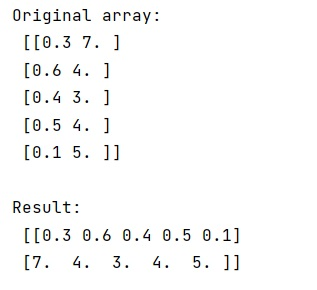# What is the equivalent of zip() in NumPy?

Learn, what is the equivalent of zip() in NumPy?
Submitted by Pranit Sharma, on February 07, 2023

NumPy is an abbreviated form of Numerical Python. It is used for different types of scientific operations in python. Numpy is a vast library in python which is used for almost every kind of scientific or mathematical operation. It is itself an array which is a collection of various methods and functions for processing the arrays.

## NumPy - Equivalent method of zip()

The zip() method returns a zip object, it runs an internal iterator where two items are paired together and the first item in each passed iterator is paired together, and then the second item in each passed iterator is paired together.

It works well with python collection data types but it doesn't seem to fit with a numpy array and hence we need to find an alternative to this method.

For this purpose, we can just transpose our array using arr.T and it will work similarly to the zip() method in the case of a numpy array.

Let us understand with the help of an example,

## Python code to demonstrate the example of equivalent method of zip()

```# Import numpy
import numpy as np

# Creating a numpy array
arr = np.array([(0.3, 7.), (0.6, 4.), (0.4, 3.), (0.5, 4.), (0.1, 5.)])

# Display original array
print("Original array:\n",arr,"\n")

# Using Transpose object as an alternative of zip
res = arr.T

# Display result
print("Result:\n",res)
```

Output: